• 而使用梅森公式，则可以不用做任何变换，只要通过对信号流图进行相应的分析就能直接写出系统的传递函数。 下面不加证明地直接给出梅森公式的定义： 计算任意输入节点到输出节点的传递函数的梅森增益公式为： P=1Δ∑...
梅森增益公式：
对于一些比较复杂的系统，采用结构图等效简化的方法求系统的传递函数是比较麻烦的。而使用梅森公式，则可以不用做任何变换，只要通过对信号流图进行相应的分析就能直接写出系统的传递函数。
下面不加证明地直接给出梅森公式的定义：
计算任意输入节点到输出节点的传递函数的梅森增益公式为：
$P=\frac{1}{\Delta} \sum_{k=1}^{n} P_{k} \Delta_{k}$
其中$\Delta$为系统的特征多项式，其计算公式为：
$\Delta=1-\sum L_{a}+\sum L_{b} L_{c}-\sum L_{d} L_{e} L_{f}+\cdots$
其中$\sum L_{a}$为所有不同回路增益之和；$\sum L_{b} L_{c}$为所有两两不接触回路增益之和；$\sum L_{d} L_{e} L_{f}$为所有互不接触的回路中，每次取其中三个回路的增益乘积之和；后面的以此类推。$n$为从输入节点到输出节点的前向通路条数；$P_{k}$为从输入节点到输出节点的第$k$条前向通路的总增益；$\Delta_{k}$为第$k$条前向通路的余子式，即把特征式$\Delta$中与该前向通道相接触的回路增益置零后剩余的部分。
举个栗子：
求下面系统的传递函数：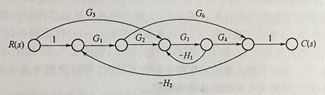先找出所有的闭环回路：
$L_{1}=-G_{1} G_{2} G_{3} G_{4} H_{2}$
$L_{2}=-G_{1} G_{6} H_{2}$
$L_{3}=-G_{3} H_{1}$
再找出两两不相邻回路：
$L_{1}$和$L_{2}$
无三个以上互不接触回路，则系统的特征式可以写为：
\begin{aligned} \Delta=& 1-\left(L_{1}+L_{2}+L_{3}\right)+\left(L_{2} L_{3}\right)=\\ & 1+G_{1} G_{2} G_{3} G_{4} H_{2}+G_{1} G_{6} H_{2}+G_{3} H_{1}+G_{1} G_{3} G_{6} H_{1} H_{2} \end{aligned}
接着找出系统的前向通路：
$P_{1}=G_{1} G_{2} G_{3} G_{4}$
$P_{2}=G_{5} G_{3} G_{4}$
$P_{3}=G_{1} G_{6}$
由于各回路均与前向通路$P_1$、$P_2$接触，故其余子式$\Delta_{1}=\Delta_{2}=1$。前向通路$P_3$不与$L_3$接触，故余子式为：
$\Delta_{3}=1-\left(L_{3}\right)=1+G_{3} H_{1}$
那么可以使用梅森公式得到系统的传递函数为：
\begin{aligned} \frac{C(s)}{R(s)}=& \frac{1}{\Delta}\left(P_{1} \Delta_{1}+P_{2} \Delta_{2}+P_{3} \Delta_{3}\right)=\\ & \frac{G_{1} G_{2} G_{3} G_{4}+G_{3} G_{4} G_{5}+G_{1} G_{6}\left(1+G_{3} H_{1}\right)}{1+G_{1} G_{2} G_{3} G_{4} H_{2}+G_{1} G_{6} H_{2}+G_{3} H_{1}+G_{1} G_{3} G_{6} H_{1} H_{2}} \end{aligned}


展开全文• 本书从函数功能、函数格式、参数说明、注意事项、Excel 版本提醒、案例应用、交叉参考7 个方面，全面、细致地介绍了Excel 2016/2013/2010/2007/2003 中公式函数的使用方法、实际应用和操作技巧。最后3 章还将公式...
• \left\{\begin{array}{ll}\delta P(R_{ij})&\text{O^i,O^j\in G^i},\\ 0&\text{otherwise}.\end{array} \right. \end{equation} 但是这样子一直编译错误，一直显示说 Missing $inserted. … 和Ex. 今天在用LATEX敲公式，想要的是这样的公式 $\rho_j= \left\{\begin{array}{ll}\delta P(R_{ij})&\text{O^i,O^j\in G^i},\\ 0&\text{otherwise}.\end{array} \right.$ 所以采用了下图的方式来实现这种 左边一个大括号，右边两行对齐的公式 \begin{equation} \rho_j= \left\{\begin{array}{ll}\delta P(R_{ij})&\text{O^i,O^j\in G^i},\\ 0&\text{otherwise}.\end{array} \right. \end{equation}  但是这样子一直编译错误，一直显示说 Missing$ inserted. …  和Extra }, or forgotten \$. … ，查了半天都没找到问题，后来突然意识到，text后面也是文字内容，如果要变成公式，需要加上$$，加上以后就正确了。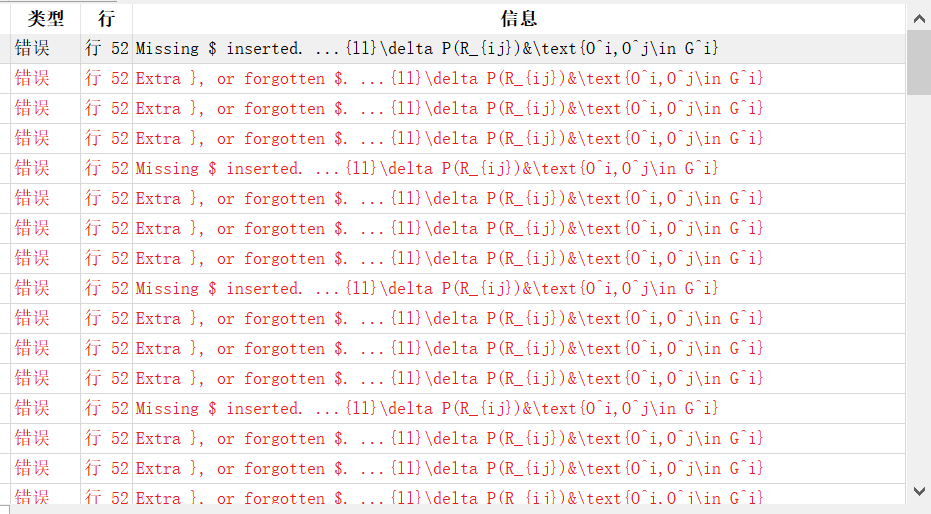修改后的： \begin{equation} \rho_j= \left\{\begin{array}{ll}\delta P(R_{ij})&\text{O^i,O^j\in G^i},\\ 0&\text{otherwise}.\end{array} \right. \end{equation}  即 $\rho_j= \left\{\begin{array}{ll}\delta P(R_{ij})&\text{O^i,O^j\in G^i},\\ 0&\text{otherwise}.\end{array} \right.$  展开全文latex • YOLO中LOSS函数的计算代码解读无目标位置confidence的delta：0 - l.output[obj_index]有目标位置confidence的delta：1 - l.output[obj_index]有目标位置坐标（x,y,w,h）的delta有目标位置class的delta代码分析和公式... YOLO中LOSS函数的计算代码解读无目标位置confidence的delta：0 - l.output[obj_index]有目标位置confidence的delta：1 - l.output[obj_index]有目标位置坐标（x,y,w,h）的delta有目标位置class的delta代码分析和公式差异二分类交叉熵求梯度关于系数 关于YOLO中LOSS函数的文章很多，GaintPanda大神最近连写三篇，这里还是从代码分析的角度再班门弄斧一下。 LOSS函数在反向传播的时候，已经分解成l.delta按batch/subdivisions进行反向传播计算了，有机会再说，这里专门扒一扒LOSS是怎么实现的。 代码解读 在darknet中，detector.c中train_detector(…)函数中训练是按批次进行的，LOSS也是按批次计算的。cfg文件中max_batches表示最大批次，单次训练使用train_detector(…)中的train_network(…)函数的执行，train_network(…)返回一个batch的LOSS值，用loss表示，并最终打印出来。 这里声明一下，讨论的batch就是cfg中配置的batch，它是一次训练图片的数量，每一次的LOSS的计算也是一个batch图片得到的结果，这一个batch的图片在load_data的时候，是从datacfg文件中所有图片中随机选出来的。实在不知道对于大规模数据集如何处理，至少目前的YOLO训练是处理中小型数据集的。 下面我们看看loss怎么计算出来的，network.c中train_network(…)代码如下： //用于CPU训练，训练需要一个network和data float train_network(network *net, data d) { assert(d.X.rows % net->batch == 0);//先对数据完整性进行验证 int batch = net->batch; int n = d.X.rows / batch;//获得子批次的个数N_eachbatch = subdivisions int i; float sum = 0; for(i = 0; i < n; ++i){ get_next_batch(d, batch, i*batch, net->input, net->truth); float err = train_network_datum(net); sum += err;//每计算一个子batch训练得到一个err，累加 } //对最终获得的误差之和求平均，分母为一个batch中的图片数 return (float)sum/(n*batch); } float train_network_datum(network *net) { *net->seen += net->batch; net->train = 1; forward_network(net);//在calc_network_cost中计算net->cost backward_network(net); float error = *net->cost;//将*net->cost复制给error //训练图片达到一个整批就可以更新一次网络参数 if(((*net->seen)/net->batch)%net->subdivisions == 0) update_network(net); return error; }  通过这个函数我们得到$LOSS=\sum_{n}^{N_{eachbatch}}cost_n$ 下面我们看forward_network(net)源代码，是如何计算每个子batch的cost的： //前向网络操作 void forward_network(network *netp) { #ifdef GPU if(netp->gpu_index >= 0){ forward_network_gpu(netp); return; } #endif network net = *netp; int i; //#pragma omp parallel for for(i = 0; i < net.n; ++i){ //每一个网络层循环一次 net.index = i; layer l = net.layers[i];//确定在哪一层 if(l.delta){ fill_cpu(l.outputs * l.batch, 0, l.delta, 1);//输出×批尺寸，统统填0 } //此处不是递归，而是一层一层的循环 l.forward(l, net);//核心代码在yolo层对应.l.type能够决定重载哪个函数原型 //计算cost，需要利用void forward_yolo_layer(const layer l, network net) //一层处理完，交给下一层，归一化层和激活层都在一个layer中，处理完，就把l.output交给net.input，开始探测下一层 //总之，只要是卷基层，操作方法都是一样的 net.input = l.output; if(l.truth) { net.truth = l.output; } } calc_network_cost(netp); }  forward_network通过l.forward(l, net)计算出l.cost，然后使用calc_network_cost对每个层的cost进行累加。所以计算的cost是三个yolo层共同的cost。通过calc_network_cost得到，得到$LOSS=\sum_{n}^{N_{eachbatch}}\sum_l^{YololayerNum}cost_l$ void calc_network_cost(network *netp) { network net = *netp; int i; float sum = 0; int count = 0; for(i = 0; i < net.n; ++i){ //如果这一层有cost，就累加，没有，就跳过 if(net.layers[i].cost){ sum += net.layers[i].cost; ++count; } } *net.cost = sum/count; }  最核心的就是每个yolo层的cost是如何计算就是计算 是通过forward_yolo_layer(…)函数中的以下语句执行的： *(l.cost) = pow(mag_array(l.delta, l.outputs * l.batch), 2);  该语句计算的是单个yolo层中所有通道feature map的元素的delta的平方，代码中用l.delta表示，元素数量为l.outputs * l.batch = l.wl.hl.n * l.batch。主要包括几类内容，序号按代码中出现的先后顺序排列： ① 无目标位置confidence的delta：0 - l.output[obj_index] ② 有目标位置confidence的delta：1 - l.output[obj_index] ③ 有目标位置坐标（x,y,w,h）的delta ④ 有目标位置class的delta LOSS函数的具体公式如下(这里引用GaintPanda中的公式)，第1、2行计算的是③项，第3行计算的是②项，第4行是①项，第5行是④项。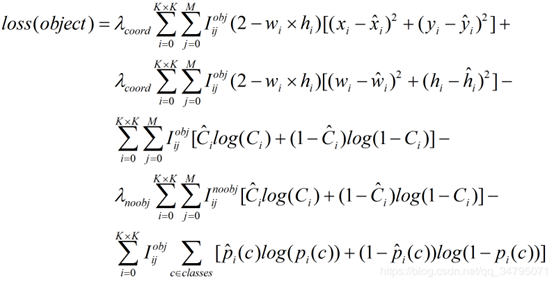下面，针对代码进行逐个讨论： 无目标位置confidence的delta：0 - l.output[obj_index] 以下是相关代码，其中b是一个subbatch中的图片数量，i,j分别是yolo层feature map的行数和列数，n为通道数。基本原理就是针对feature map每个位置的坐标和长宽的预测值，和相应图片的GT参数进行遍历比较，如果IOU大于l.ignore_thresh（在cfg文件中为0.7），就认为是有目标，将其忽略掉。计算剩下的位置的confidence值的delta，即l.delta[obj_index] = 0 - l.output[obj_index]。目标是将 l.output[obj_index]降为0. 注意，这里没有判断confidence有没有达到某个阈值，而是使用IOU来判断。使用全体位置的confidence减去真值位置的confidence是比直接计算无目标位置的confidence有效的，因为刚开始训练的时候，IOU应该都很小，甚至刚开始的时候全都是无目标，随着IOU逐渐增大，渐渐抛去无目标点的值，这样可以尽可能网罗所有的无目标点。如果使用IOU小于某个阈值为无目标、剩下的都是有目标的策略可能导致虚警率偏高。 int box_index = entry_index(l, b, n*l.w*l.h + j*l.w + i, 0);//获得正在处理的预测框的中心点的索引 //对于同一类目标，认为预测框的基本形状应该是相同的：l.mask[n]，或者之前的猜测并不正确，输出分为三大块只是为了针对不同的先验框 //因为相对尺寸只有针对固定的先验框才有意义。同时，如果重合点上有不同的目标，也是可以识别出来的 //重合点上甚至可以有多个相同类型目标，重合点上的多个相同类型目标如果需要识别出来，必然形状是不同的，否者不就遮挡了吗 //相信两个长宽相同的不同目标重叠在一起识别率应该非常低 //mask[n]对应第n个YOLO输出块，pred是预测的结果 box pred = get_yolo_box(l.output, l.biases, l.mask[n], box_index, i, j, l.w, l.h, net.w, net.h, l.w*l.h);//获取预测框 float best_iou = 0; int best_t = 0; //注意，这里是每个feature map上的点都和所有的label对应一遍，这个对应也只和训练有关，因为如果是非训练的情况 //根据GT和pred的IOU，选出IOU最大的，认为如果大于l.ignore_thresh，就认为命中目标，其余的都要当成No Obj计算delta for(t = 0; t < l.max_boxes; ++t){ //每检测到一个box,就对照数据集，把正样本（目标）的坐标都对比一遍，计算IOU //倒着加数量(GT的指针+图片序号×GT个数+具体的位置)，net.truth是所有truth位置的起点 box truth = float_to_box(net.truth + t*(4 + 1) + b*l.truths, 1);//这里面的truth是box，1为步长 if(!truth.x) break;//如果没有找到标签框就退出，或者说一个sub批次对应的标签数据循环完就退出 float iou = box_iou(pred, truth);//计算IOU,更新最新的IOU值，然后确定哪个正样本和测试集一致 if (iou > best_iou) { best_iou = iou;//如果最新的IOU最大，则选择这个最好的先验框作为匹配框 best_t = t;//注意，这表明每个图中不一定只有一个目标，训练集也不会提供那么优越的条件，所以要在图像里面找到IOU最一致的认为是目标，这种方法有风险。这个t是针对feature map上一个目标点的结果。 } } int obj_index = entry_index(l, b, n*l.w*l.h + j*l.w + i, 4);//选择confidence,即第5个框 //可信度的值加到avg_anyobj参数上，先将所有的位置的confidence都加起来 //No Obj和Obj是两个不同的概念，No Obj是所有目标的confidence低的，Obj是确实有目标的 avg_anyobj += l.output[obj_index]; //可信度的delta为0-l.output[obj_index],用0做减法，就可以使用交叉熵，不管用不用都不影响 l.delta[obj_index] = 0 - l.output[obj_index]; //如果最好的IOU＞忽略delta的阈值，则delta=0，表示默认这个点是真值,delta是0不意味着l.output[obj_index]==1 //cfg中ignore_thresh设置为0.7，不苛刻，这里判断先验框和真值。//0524，下午再研究前半段和后半段区别 if (best_iou > l.ignore_thresh) { l.delta[obj_index] = 0; }  有目标位置confidence的delta：1 - l.output[obj_index] 代码如下，基本原理是先对比先验框anchors和GT的IOU，确定先验框和选择在第几个feature map目标数据块中，因为先验框musk定了，在第几个目标数据库也确定了，就认为这个位置是有目标的，然后计算每个有GT且先验框正确的位置上的confidence的delta = 1 - l.output[obj_index]。这个有GT的位置在张量上的坐标为（i,j,mask_n*255+4）。 这时候就有一个问题了，前面计算的无目标点会和后面的有目标点重合吗？当然会啦！但是这并不影响，我们针对这几种目标逐个分析： a）当feature map这个位置本来没有目标，同时也每检测到目标，计算0 - l.output[obj_index]，然后调整weight降低这个值，可以减少无目标点的虚警。 b）当feature map这个位置本来有目标，同时又预测到（best_iou>0.7）时，计算1 - l.output[obj_index]，然后调整weight降低这个值，可以提升有目标点的准确率。 c）当feature map这个位置本来有目标，但又没有预测到（best_iou≤0.7）时，先令l.delta[obj_index] = 0;然后再计算l.delta[obj_index] = 1 - l.output[obj_index]，然后仍然是提升有目标点的准确率。 //l.biases的值为cfg文件中anchors for(t = 0; t < l.max_boxes; ++t){//遍历每个GT,检验每个框的尺寸是不是最准的，这个地方抛弃了(x,y) box truth = float_to_box(net.truth + t*(4 + 1) + b*l.truths, 1); float best_iou = 0; int best_n = 0; i = (truth.x * l.w);//feature map中真值的点位，注意，label中的坐标点为归一化的点，便于在程序中比较 j = (truth.y * l.h); box truth_shift = truth; truth_shift.x = truth_shift.y = 0;//预选框的坐标点归零 //在每个预测框的位置，检验所有可能的目标，判断相同位置最准确的那个目标，yolo层total应该为9 //这里采用了预置框进行训练，total为预置框的个数，l.total = 3，目标选出best_n for(n = 0; n < l.total; ++n){ box pred = {0}; pred.w = l.biases[2*n]/net.w; pred.h = l.biases[2*n+1]/net.h; float iou = box_iou(pred, truth_shift); if (iou > best_iou){ best_iou = iou;//如果iou＞0，就保留该序号，这个地方是为了找到正确的预选框，注意预选框和GT可不是一回事哦 best_n = n; } } //挑一个真值和预选框近似的，三个尺度不同长宽，总有一款适合你 //在l.mask中，如果有和最佳预置图片序号相等的，返回mask的序号，没有的话返回-1 //默认，同一个GT不可能落入相同的musk中 int mask_n = int_index(l.mask, best_n, l.n); //下面的操作都是针对每个GT位置上的 if(mask_n >= 0){ //box index落在了第几个目标块中，通过真值锁定，不可能出现一个真值在相同的位置的不同的目标快中都有标记 int box_index = entry_index(l, b, mask_n*l.w*l.h + j*l.w + i, 0); //注意，这里是关键，delta_yolo_box,计算iou和delta，这里只计算box_index位置有没有目标，和真值差多少，及IOU float iou = delta_yolo_box(truth, l.output, l.biases, best_n, box_index, i, j, l.w, l.h, net.w, net.h, l.delta, (2-truth.w*truth.h), l.w*l.h); //返回目标点confidence的index int obj_index = entry_index(l, b, mask_n*l.w*l.h + j*l.w + i, 4); avg_obj += l.output[obj_index];//获得和真值重合的位置的均值 l.delta[obj_index] = 1 - l.output[obj_index];//计算目标点上的Obj的误差 int class = net.truth[t*(4 + 1) + b*l.truths + 4];//获得真值类型的标签 if (l.map) class = l.map[class];//没有mapfile，l.map就置0，这条不执行 //获取类型的index位置，class已经确定，这个index是读取内存的位置 int class_index = entry_index(l, b, mask_n*l.w*l.h + j*l.w + i, 4 + 1); delta_yolo_class(l.output, l.delta, class_index, class, l.classes, l.w*l.h, &avg_cat); ++count;//目标的个数 ++class_count;目标class的个数，每运行一次加1，参与每个批次的大循环累加 if(iou > .5) recall += 1;//真值点检测出来目标iou＞0.5的个数，所有真值中，检测到了，查漏 if(iou > .75) recall75 += 1;//真值点检测出来目标iou＞0.75的个数，根据实际数据，＞0.5容易，甚至可以达到0.9以上，＞0.75不易 avg_iou += iou;//计算平均IOU } }  有目标位置坐标（x,y,w,h）的delta 这里利用forward_yolo_layer(…)中的下面代码进行计算： float iou = delta_yolo_box(truth, l.output, l.biases, best_n, box_index, i, j, l.w, l.h, net.w, net.h, l.delta, (2-truth.w*truth.h), l.w*l.h);  我们看看delta_yolo_box(…)的源码，这个原理是将GT值变成和预测值对应的值，然后相减。GT值和预测值存储的都是一个相对值，具体这几类值之间的不同及边框预测的内容将在后面专门写。这里挖个坑，后面将专门写一篇讨论图片从进入网络各种尺寸变化和边框预测的文章，同时也将会聊一聊和目标预测相关的变尺度训练。大体原理先看本文源码注释。 这里没有把图片真实的结果算出来再相减，全都使用相对值、偏置值，是为了归一化delta，避免出现因为尺度不同导致delta剧烈波动从而使得weight预测难以回归。 //参数： //输入真值truth，data数据x,偏置biases，预选框编号n //输出平面上有真值的序号index，真值在feature map中的位置坐标i,j,feature map的宽度lw和高度lh,网络输入层的宽度和高度 //误差delta,缩放尺度scale = 1，步幅stride = 1 //计算出来了index位置的值，其他位置赋值为0 float delta_yolo_box(box truth, float *x, float *biases, int n, int index, int i, int j, int lw, int lh, int w, int h, float *delta, float scale, int stride) { box pred = get_yolo_box(x, biases, n, index, i, j, lw, lh, w, h, stride);//获取预测值 float iou = box_iou(pred, truth);//计算iou //使用GT坐标（相对值）乘以feature map长宽，减去真值的位置，得到GT在feature map中坐标的偏置 float tx = (truth.x*lw - i); float ty = (truth.y*lh - j); //计算GT和先验框的宽度缩放比值，取log float tw = log(truth.w*w / biases[2*n]); float th = log(truth.h*h / biases[2*n + 1]); delta[index + 0*stride] = scale * (tx - x[index + 0*stride]);//计算GT和预测值的差，GT在feature map中坐标的偏置减去预测到的偏置 delta[index + 1*stride] = scale * (ty - x[index + 1*stride]); //计算GT宽高和预测值相应值的误差 delta[index + 2*stride] = scale * (tw - x[index + 2*stride]); delta[index + 3*stride] = scale * (th - x[index + 3*stride]); return iou; }  有目标位置class的delta 这里利用forward_yolo_layer(…)中的下面代码进行计算： delta_yolo_class(l.output, l.delta, class_index, class, l.classes, l.w*l.h, &avg_cat);  源码如下。原理为：最初，所有的delta都是0，则进入for循环遍历每个class，在非GT的class的位置，统统计算delta = 0-l.output[index + striden]，在GT的class的位置，计算delta = 1- l.output[index + striden]。 //计算yolo中class的误差 //输入为YOLO层的输出，类型的初始位置index，GT类型class，类型数classes，步幅stride，*avg_cat为积累输出 //当令输入avg_cat = 0，永远不会积累 //当令输入avg_cat 为参数输出，就不停的积累下去 void delta_yolo_class(float *output, float *delta, int index, int class, int classes, int stride, float *avg_cat) { int n; //如果这个输出点的误差不为0，操作完if就返回，否则执行for循环。 //一次输出积累都没有的时候，全都赋值0，去进行下面的循环，得到delta值。在index上输出的delta != 0,计算一次*avg_cat，表示catch到一个目标类型 //接着开始不断在class层计算强化 if (delta[index]){ delta[index + stride*class] = 1 - output[index + stride*class];//第class个输出平面的第index位置的误差 = 1-相同位置输出值 if(avg_cat) *avg_cat += output[index + stride*class];//如果avg_cat指针不为空，就不断的进行输出值积累 return; } //遍历每个类型，即搜索每个输出平面 for(n = 0; n < classes; ++n){ //在非GT的class的位置，统统计算delta = 0-output，在GT的class的位置，计算delta = 1- output。 delta[index + stride*n] = ((n == class)?1 : 0) - output[index + stride*n]; //如果在class这一层，avg_cat≠0，说明输出是正确的，则继续在avg_cat上积累输出 if(n == class && avg_cat) *avg_cat += output[index + stride*n]; } }  但是这一切都有个前提：针对于同一张图片中的目标，class从label中读取，放在对应的feature map中，GT坐标点(truth.x,truth.y)虽然各不相同，但是计算出来的(i,j)有量化误差，导致相同的i,j可能有多个目标。由于计算delta针对每一个GT进行遍历的，当两个目标落入一个(i,j)，对应的index相同，第二次计算delta[index]时，delta[index]已经不为0了。怎么办？如果再进入遍历每个class的for循环，就会把之前计算的结果覆盖掉。对于同一张图片，l.output还是那个l.output，如果是非class那个位置，还是等于delta = 0-l.output[index + striden]，没必要重新计算一遍。现在作者想在同一个位置上保留两个GT的class的delta，就只需要把class_new位置的delta更新一下返回即可。于是就出现了if (delta[index])判断的过程。 因为分类的时候使用的logistics激活函数(sigmoid)，在相同的feature map的输出点（位置）上对不同class的位置（i,j,255l.n+4+class）上的计算都是独立的，很可能导致在探测的时候就将一个目标预测成多个类型的情况，训练过程中也支持这种事情的发生。解决的办法可以在输出探测结果时对获得的类型进行排序呢，选取概率最大那个，亲测还是比较准的。如果将sigmoid换成sofxmax，在训练时候包容性就会降低，会导致预测分类准确度降低。 代码分析和公式差异 根据前面LOSS函数的具体公式，我们看到confidence和class的loss计算使用的是交叉熵，可是代码一看就是平方根。难道有什么错吗？BBuf的两篇文章《你对YOLOV3损失函数真的理解正确了吗？》和《YOLOV3损失函数再思考 Plus》进行了详细的说明。根据文章我们可以这样理解，LOSS函数的计算首先是为反向传播求梯度服务的，而通过求导我们发现交叉熵和平方根的计算结果是一样的，那么我们就可以认为这个程序使用的是交叉熵。当然并不是所有的模型交叉熵和平方根求梯度效果一样，只不过这里使用的是二分类。本文作为学习笔记将对这个问题再推导一遍。 二分类交叉熵 交叉熵来源于KL散度，理解二分类交叉熵，我们先说一个词KL散度。$\hat{c}_i$表示样本的真实概率，这个是客观存在的，是我们希望通过学习训练逼近的。${c}_i$表示样本的预测概率，是通过不断训练才能得到的。KL散度是对真实分布和预测分布两种不同的概率分布差异的度量，公式如下：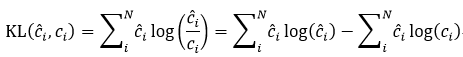KL散度很好理解，公式中$-log({\hat{c}_i})$⁡(就是真实分布的信息量，$-log({\hat{c}})$⁡就是预测分布的信息量，KL散度就是计算真实分布和预测分布信息量的差值，再对这个差值求期望，得到一个真实分布和预测分布的差熵。 进一步展开得到上面公式的第二个等号内容，等号右边第一项表示真实分布的信息熵取反，是固定不变的，剩下一部分就是交叉熵，可见交叉熵和KL散度的效果是一样的。这个和互相干有点类似。交叉熵公式如下：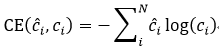二分类交叉熵即binary cross entropy，简写为BCE，计算公式如下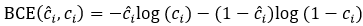这是什么意思呢？对于二分类模型来说，存在有目标（obj）和无目标（noobj）两种情况，$BCE(\hat{c}_i,{c}_i)$表达式的两项分别就对应着两种情况。二分类交叉熵就是是与不是两种情况的交叉熵，有木有变得很简单了？ 在YOLO模型计算confidence和class的时候，计算得到的都是概率，所以l.output的输出可以直接带入交叉熵LOSS函数。由于使用的是二分类模型，激活函数使用的也是sigmoid，confidence计算的结果是有没有目标，class计算的结果是是不是这个类型的目标。如果是采用softmax分类函数的话，则需要使用正常的交叉熵公式进行计算。 求梯度 好了，说完了二分类交叉熵的概念，我们怎么计算LOSS呢？前面说了，由于MSE和BCE求梯度结果相同，作者使用MSE对BCE进行替代计算。我们一起来推导一下$\frac{∂LOSS_c}{∂z_j}$，求出这个就可以进行反向传播，$z_j$是卷基层feature map最后一层的l.output。这里我们只计算confidence和class的，由于原理相同，我们用$LOSS_c$表示。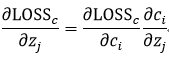看前半部分$\frac{∂LOSS_c}{∂z_j}$，对于$CE(\hat{c}_i,{c}_i)=-\sum_i^{N}{\hat{c}_ilog({c}_i)}$,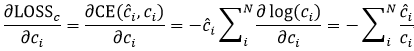对于$BCE(\hat{c}_i,{c}_i)$，如果$\hat{c}_{obj}=1$，则$\hat{c}_{noobj}=1-\hat{c}_{obj}=0$，$BCE(\hat{c}_i,{c}_i)=-log⁡(c_{obj})$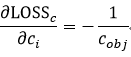再对后半部分求导，先计算softmax的激活函数$c_i=\frac{e^{z_{j} }}{\sum_k{e^{z_k}}}$的求导，再简化为sigmoid激活函数$c_i=\frac{1}{1-e^{z_{j} }}$ 的求导。 对于softmax的激活函数$c_i=\frac{e^{z_{j} }}{\sum_k{e^{z_k}}}$，关于$z_j$的偏微分为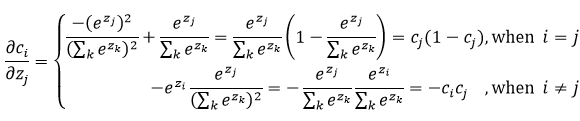对于sigmoid激活函数$c_i=\frac{1}{1-e^{z_{j} }}$ ，当$i≠j$，$\frac{1}{1-e^{z_{j} }}$ 对$c_i$于来说就相当于常数，关于$z_j$的偏微分为： 接下来我们开始组合。首先是多分类交叉熵CE：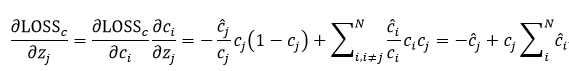对于二分类交叉熵BCE，$\sum_i^{N}\hat{c}_i =1+0=1$，可简化为：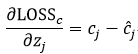那么对于多分类交叉熵是否存在$\sum_n^{N}\hat{c}_i=1$答案是否定的。其实对于$\hat{c}_j$来说，对于确定的GT，feature map上对应的概率就是1或者0，而我们训练的时候只不过使用一个概率去逼近，输出的就不可能100%是1或者0，如果是的话，那么泛化能力就没有了。所以甚至可以直接写出来$\frac{∂LOSS_c}{∂z_j }=c_j-1$，这个c_j表示预测的有目标时候的概率。对于noobj，$(∂LOSS_c)/(∂z_j )=c_j-1$。关于正负号，做平方以后效果是一样的。 对于MSE呢？$LOSS_c=\frac{1}{2}(\hat{c}_i-c_i)^2$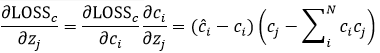显然对于多分类交叉熵和MSE求导是不一样的，但是对于二分类呢？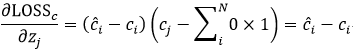可以看到，BCE和MSE求导是相同的，所以我们可以认为LOSS函数在求confidence和class的时候使用的就是二分类交叉熵。 在《YOLOV3损失函数再思考 Plus》中，作者说box的参数（x,y,w,h）都使用了sigmoid函数，但是仔细看程序： for (b = 0; b < l.batch; ++b){ for(n = 0; n < l.n; ++n){ int index = entry_index(l, b, n*l.w*l.h, 0); activate_array(l.output + index, 2*l.w*l.h, LOGISTIC); index = entry_index(l, b, n*l.w*l.h, 4); activate_array(l.output + index, (1+l.classes)*l.w*l.h, LOGISTIC); } }  这里只针对x,y使用了sigmoid函数，本人认为计算x,y使用sigmoid函数是通过激活函数吧l.output限制在[0,1]的区间中，就好像计算w/truth.w的时候加了log再输出是为了避免梯度爆炸或者梯度退化采取的一种数学手段。 关于系数 我们看到公式中有两个系数$λ_{coord}$和$λ_{noobj}$，但是在程序代码中完全没有出现。其实查看YOLOv1和YOLOv2的cfg文件时候可以看到，原来是存在的，而且都不是1。这个系数可以理解为x,y,w,h,confidence,class的loss分项的权重，通过这个参数来控制不同loss的影响力，但是在YOLOv3中全都删除了，可能是这个参数影响真的没有那么大。 另外在x,y,w,h相关的loss函数项中，还有一个系数$(2-w_i×h_i)$，这个是用于解决当目标过小的时候，对其loss进行一个比例放大，从而减小训练误差。对于$w_i,h_i＜1$，如果$w_i,h_i$越小，$(2-w_i×h_i)$越大，这部分权重就越大，误差产生的影响就可以进一步放大，有人做实验针对 YOLOv3，如果不减去$w×h$，AP 会有一个明显下降，如果继续往上加，如 $(2-w_i×h_i)×1.5$，总体的 AP 还会涨一个点左右（包括验证集和测试集）。  展开全文深度学习 人工智能 • P=\frac{1}{\Delta}\sum\...P：传递函数 n：前向通路总数 前向通路：从输入到输出，每个节点只经过一次。 \Delta：流图特征式，1-\sum L_a + \sum L_bL_c - \sum L_dL_eL_f + \ldots \sum La... P=\frac{1}{\Delta}\sum\limits_{k=1}^np_k\Delta_k P：传递函数 n：前向通路总数 前向通路：从输入到输出，每个节点只经过一次。 \Delta：流图特征式，1-\sum L_a + \sum L_bL_c - \sum L_dL_eL_f + \ldots \sum La：所有单独回路增益之和 \sum LbLc：两两不接触的回路增益之和 \sum L_dL_eL_f：三三不接触的回路增益之和 p_k：第k条前向通路增益 \Delta _k：流图余因子式，等于流图特征式中除去与第k条前向通路增益相接触的回路增益项之后的余项 下面是一个例子。单独回路：\sum L_a = -G_1 - G_2 - G_3 - G_1G_2 两两不接触回路（直接从上面的单独回路里挑选）：\sum L_bL_c = G_1G_2 + G_1G_3 + G_2G_3 + G_1G_2G_3 三三不接触回路：\sum L_dL_eL_f = -G_1G_2G_3 于是流图特征式\Delta = 1+ G_1+G_2+G_3+2G_1G_2+G_1G_3+G_2G_3+2G_1G_2G_3 各前向通路以及余因子式： p_1=G_1G_2G_3K，\Delta _1 = 1 （从\Delta中删除包含有p_1各乘积项的因子即得到\Delta_1） p_2=G_2G_3K， \Delta _2 = 1+G_1 p_3=G_1G_3K, \Delta_3=1+G_2 p_4=-G1G2G3K, \Delta_4=1 于是传递函数为： \frac{C(s)}{R(s)}=\frac{p_1\Delta _1 + p_2\Delta _2 + p_3\Delta _3 + p_4\Delta _4}{\Delta} =\frac {G_2G_3K(1+G_1) + G_1G_3K(1+G_2)} {1+G_1+G_2+G_3+2G_1G_2+G_1G_3+G_2G_3+2G_1G_2G_3}  展开全文• Delta”是一个专门用于不确定性的符号和数值计算的函数。 不确定性计算基于用户定义的函数的偏导数的符号评估。 通过对变量不确定性进行偏导数加权来获得不确定性向量的范数，可以使用以下公式执行： - L1 范数：...matlab • 导数与微分，导数的计算知识点微分的定义导数运算法则基本初等函数导数公式变限积分求导参数式、隐函数、幂指函数、反函数 求导解题思路2.导数的应用知识点相关定义凹凸性判断拐点的条件渐近线求法曲率解题思路3.... • ## SVM损失函数 千次阅读 2017-12-16 22:01:47 SVM（支持向量机）和softmax的区别在于损失函数的不同，用公式可以描述为： Li=∑j≠yimax(0,sj−syi+Δ)Li=∑j≠yimax(0,sj−syi+Δ)L_i=\sum_{j\neq y_i}max(0,s_j - s_{y_i}+\Delta) 其中syisyis_{y_i} 表示...svm 正则化 • ，forward函数一直在计算l.delta，backward函数也是和l.delta有关系的，看l.delta的计算方式，怎么看都不像是loss的公式，那么，l.delta究竟是什么，搞明白这个问题需要了解反向传播的原理，由于pytorch已经将反向...神经网络 • （由于不方便上传公式，有部分直接截图）2. Polyval函数对应多项式拟合，多项式求值函数也相当重要，可以通过设定向量，...S即为polyfit函数返回的误差估计，具体delta的数学意义本人暂时不清楚 y = polyval(p,x,[], • 文章目录#傅里叶变换##基本性质##drac−deltadrac-deltadrac−delta函数（单位冲激函数）##卷积#LaplaceLaplaceLaplace变换##基本性质##卷积##公式大全##求拉氏逆变换###公式法###留数法 欢迎纠错 #傅里叶变换 ##...卷积 傅里叶变换 拉普拉斯变换 积分变换 • #!...#公式总结 物理量 公式 条件 ...δW=def−pexdV\delta W\overset{def}{=}-p_{ex}dVδW=def−pex​dV ...UUU U是状态函数 ΔU=Q+W\Delta U=Q+WΔU=Q+W 热力学第一定律表达式，任何情况都可以用 Q • 基础教学和快速参考 函数显示方式 内联函数（插入）...表示......... 显示函数（居中）$$...$$表示......... ...小写： \alpha \beta...大写：$$ \Alpha \Beta \Gamma \Delta \Omega表示为ABΓΔΩ \Alph...MARKDOWN KaTex 基础 • YOLOV3-损失函数及其源代码理解（yolo_layer.c） 讲得好 https://github.com/AlexeyAB/darknet/issues/821 x,y,w,h 损失 原版的bbox损失为MSE，其后又GIOU，DIOU… delta即为求完损失的梯度 公式对应图 思路及具体... • 因为傅里叶变换之类的很常用，时间长了不用总会忘记，所以一次性罗列出来权当总结好了。主要参考《信号与线性系统分析》(吴大正)，也有的部分参考了复变函数。...一阶导数和函数的乘积\[f(t)\delta'(t-t_0)... • 广告系统智能出价PID控制中的偏差函数主要有差分形式和比率形式，响应函数有线性响应和非线性响应。 根据具体的场景、目标的不同，可以自己对偏差函数...我们知道控制出价的公式为 ecpm=1000∗pctr∗bidcpc ecpm = 1000推荐系统 深度学习 算法 广告 机器学习 • 神经网络初探：反向传播公式的简易证明​ 我们已经知道反向传播算法的核心在于求解代价函数对各个参数的偏导，由此我们需要四个公式，前两个公式让我们能够求解每一层的误差δ\delta（errors）,后两个公式给出偏导数...神经网络 数学证明 反向传播算法 • 使用该公式不用判断象限，直接得出方位角值...\Delta Y=\Delta Y+1^{-10}  为了使除数不为零而加一个很小的数(注：在参与计算前加一个极小值后参与sign函数计算，这样sign(ΔY\Delta YΔY)就只会出现1或-1两种情况... • 使用aligined 函数，其中aligned就是用来公式对齐的，在中间公式中，\ 表示换行， & 表示对齐。在公式中等号之前加&，等号介绍要换行的地方加\就可以了。 \begin{equation} \begin{aligned} L_{task} = &...latex • 之后通过自己手动推导系统传递函数与阶跃响应函数公式，并绘制时间域关于 ttt 响应曲线，从而验证自己推导的有效性。 sys1 = tf(,); subplot(2,2,1);step(sys1);title('F(s) = 1 ---> f(t)=delta(t)'); ...算法 人工智能 计算机视觉 matlab • 是关于所有智能体策略参数 \left(\boldsymbol{\theta}^{1}, \boldsymbol{\theta}^{2}\right) 的函数。NL 通过以下迭代过程不断更新两个智能体的参数： \begin{array}{l} \boldsymbol{\theta}_{i+1}^{...
• 自相关性 求取滑动窗口内的自相似性，用自相关函数表示： ... \Delta x, \Delta y)=\sum w(x, y)(I(x, y)-I(x+\Delta x, y+\Delta y))^{2} c(x,y;Δx,Δy)=∑w(x,y)(I(x,y)−I(x+Δx,y+Δy))2 其中III...矩阵
• 用maclaulin公式计算arcsin(x) ：x+x^3/(2*3)+1*3*x^5/(2*4*5)+...+(2n)!*x^(2n+1) / [2^(2n)*(n!)^2*(2n+1)]。 用户输入x，利用该式，计算反正弦函数的值。当最后一项小于delta时停止计算,输出结果。其中，^...python
• 公式：ρ=1−6∑i=1nΔri2n3−n\rho=1-\dfrac{6\sum\limits_{i=1}^n\Delta{r_i}^2}{n^3-n} 其中nn是每组数据的个数；Δri\Delta{r_i} 是对应的第ii对数据在各自数组中的次序之差；因为次序rr的定义有不同可能，所以...vba excel spearman 相关系数
• 函数deltaE = sRGB2CIEDeltaE(SamplesRGB1,SamplesRGB2,公式) 计算一组 sRGB 数据对 SamplesRGB1 和样本RGB2（均在[0 1]内）。 CIEDE1994、CIEDE2000和CIELAB可根据用户输入选择公式，可以是“cie94”、“cie00”和...matlab
• SFT=2πδ~(ω)2\pi\tilde \delta (\omega )2πδ~(ω)，其中δ~(ω)\tilde \delta (\omega )δ~(ω)为以2π2\pi2π为周期的周期单位冲激函数。 SFT[ejω0n{e^{j{\omega _0}n}}ejω0​n]=2πδ~(ω−ω0)2\pi\...数字信号处理 功率谱密度 物理意义 能量信号 功率信号
• matlab中inv的函数代码近似黑森州 本课程的目标是针对太阳黑子数据训练不同的网络并比较结果。 首先，已计算出作为...最后，使用以下公式更新权重： W i+1 = W i + Inv_H(a,a) * delta_weights(a) Inv_H对应于先前计